The First Personality Reading Based On
The Sacred Geometry Of Your Name Symbols.

# From Numerology to Geonumerology

From a linear & monolithic approach of personality
to a ternary holistic vision of human being### The ancient numerology of Pythagoras

Numbers, symbols and ideas

Geonumerology is an interdisciplinary approach which aims to restore the ancient numerology of Pythagoras to its former glory. It is therefore at the heart of the teaching of this philosopher that Geonumerology finds its source. Arithmetic and geometry were, along with astrology and music, the four sciences that were taught at the time within the framework of the Quadrivium.

Pythagoras is certainly the first great philosopher of the Western tradition. It was he who invented the word philosophy. Everyone knows Pythagoras for his multiplication tables or his theorem. But what is less known is that his mathematics actually leads to a metaphysics, being convinced that numbers are the principle and the key to the entire universe.

His main idea is to have considered the universe as an ordered whole, a "Cosmos", where the numbers serve as a support for a vibratory reality. We can therefore legitimately consider Pythagoras as the father of current physics.

As Man, or microcosm, is the image of the universe, the macrocosm, the laws that apply to one also apply to the other.

The initiatory process which characterizes his teaching is related to the realisation of the Self in psychology as well as to the quest for the philosopher's stone of the alchemists. These three paths invite us to direct our gaze inward, in a process of introspection to the discovery of our soul.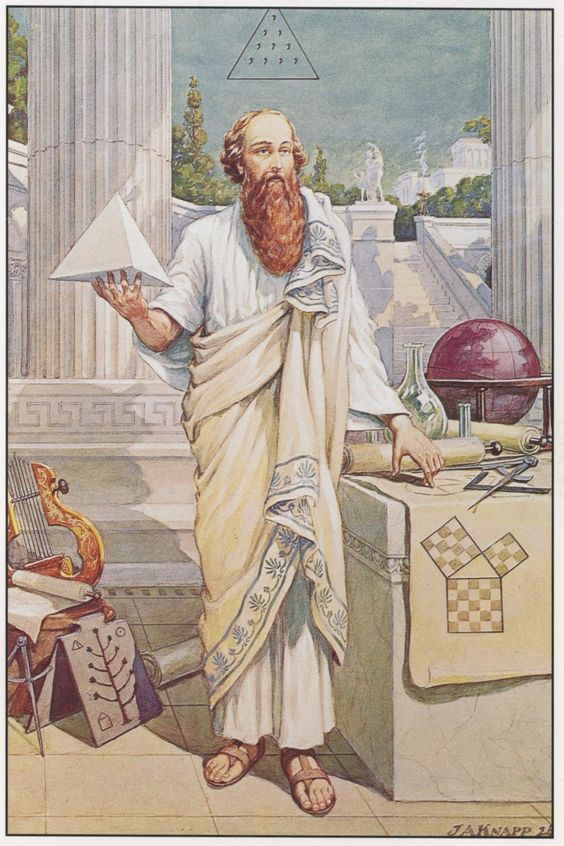## Analogy and correspondences

The number and its representations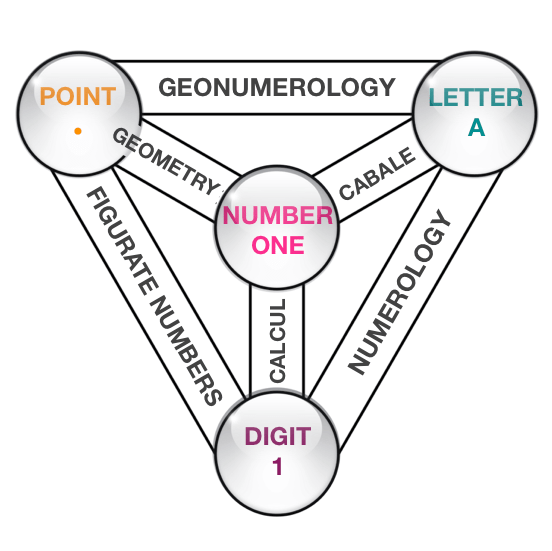### Law of Analogy

#### ONE = •

Man learned to count long before he could write. Using notches in bones or wood and then small pebbles, from the Latin calculi, plural of calculus.

#### ONE = A

Then with the arrival of writing, letters were then used to designate numbers. This is notably the case of the Hebrew and Greek alphabets. The number 1 is thus associated with the letter Alpha in Greek or Aleph in Hebrew.

#### ONE = 1

The spelling of the numbers (1,2,3, ...) that we use for the calculation, is of Indian origin and was transmitted to Western civilisation by the Arabs in the 10th century.

### Theory of correspondences

#### If A = 1

Each letter has a numerical value

This is principle of Numerology.

#### And 1 = •

To the arithmetic unit unit corresponds the geometrical point.

This is the principle of geometry.

#### So A = •

It is therefore possible to to visualize any word or Name according to the number of units it contains.

Geonumerology synthesis

"We must not confuse Numbers, which are Ideas-Strengths, intermediaries between the Visible Plane and the Invisible Plane, and Digits, which are the clothing of numbers. »

The Science of Numbers
by Papus (Dr Gérard Encausse) - 1934
Posthumous work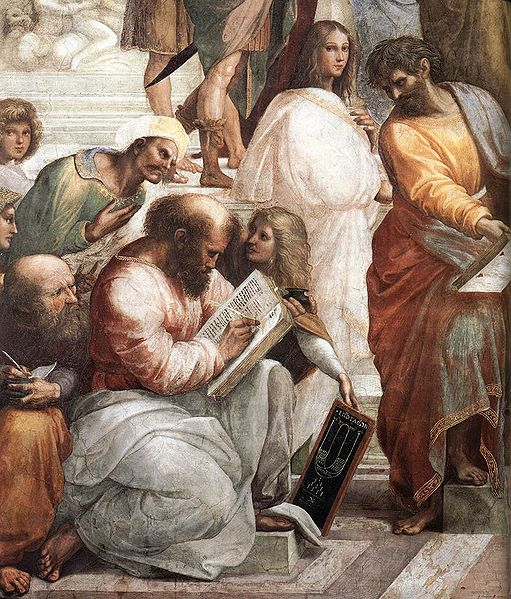## Analogy between letters and numbers

Principle of numerology

#### The number of your name

Numerology takes up the teachings of Pythagoras and associates a numerical value to each letter: 1 for A, 2 for B, 3 for C, etc.

For numbers with more than one digit, we apply theosophical reduction which adds up the digits of the number so that only one remains. Ex: J = 10 = 1 + 0 = 1, K = 11 = 1 = 1 = 2, etc.

Thus it is possible to calculate the Number of your Name, by adding up the letters in it, and then, by reducing the total, to find a number between 1 and 9.

"The Pythagoreans assured that each letter has a certain number which indicates the future; that one can guess what must happen to men by calculating the numbers contained in the letters of their proper names. »

Agrippa - De Vanitate Scientiarum

### Correspondences between letters and numbers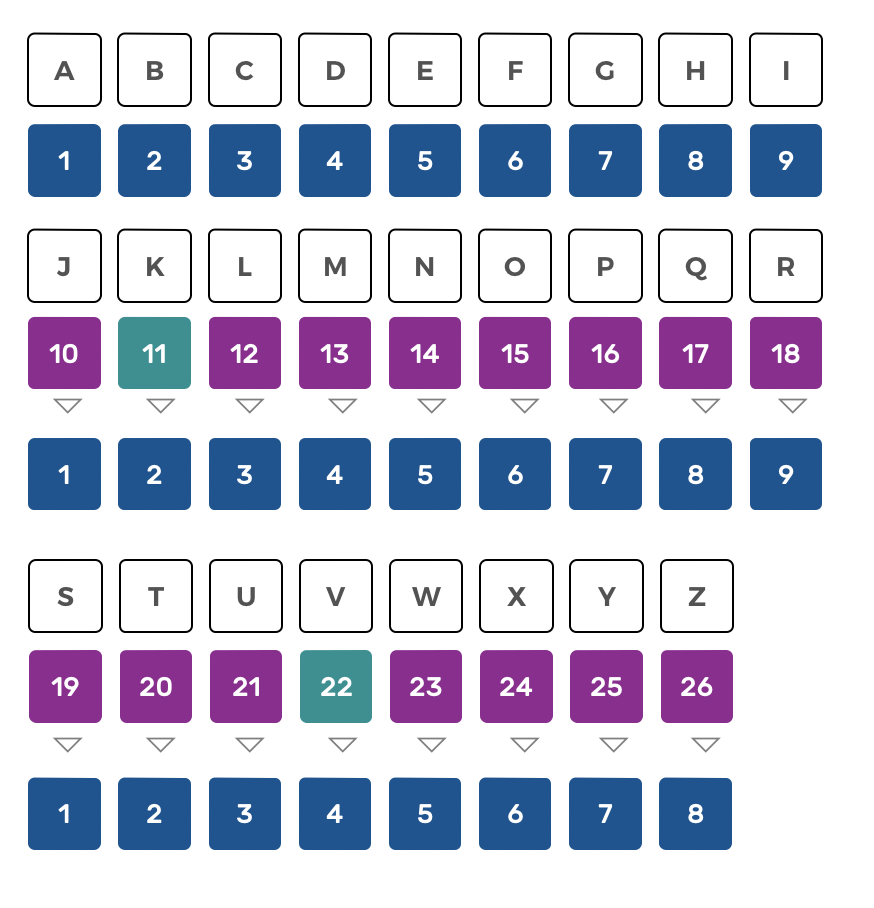Contemporary numerology does not practice reduction for the numbers 11 and 22, considering them as master numbers. We will see below whether this makes sense or not.## Alpha-numeric table

Write your LAST NAME and FIRST NAME in capital letters and then indicate just below the value of each letter, using the mnemonic matrix.

Then add up the total until you get a number between 1 and 9. Remember to memorize the last operation that precedes the reduced number. For example 4 obtained by 1 + 3, 2 + 2 or 4 + 0.

Man receives a name at birth, which will distinguish him from others but above all characterizes his Personal Vibratory Signature. This vibration varies for each being according to the frequency and, evolves in time. To know the name of an individual, of a thing, is to know his being. The Name has therefore hugely importance, because by its vibrations it expresses the essence of a being or an object.

## Master numbers or selfish numbers

Let's have a look inside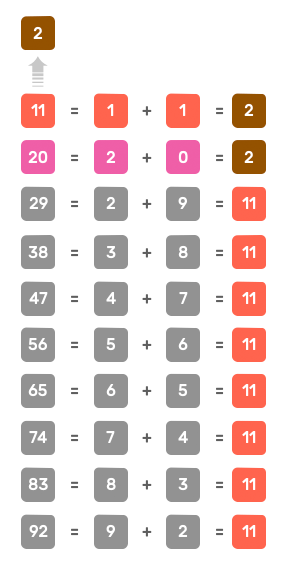Of the ten decompositions identified above, only one concerns the number 20 and nine concern the number 11, i.e. 90% of the cases.
In other words, all the 2 are 11 !

#### Master number 11

Modern numerology distinguishes two cases of what it calls ‘Master Numbers’ : 11 and 22, which are not subjected to reduction. Pythagoras considered these dual numbers to be egotistic, as they were formed from a single number that is paired with itself.

Additionally, this designation as ‘Master Numbers’ seems largely exaggerated, as the number 2 is obtained 90% of the time from 11 (29, 38, 47, 56, 65, 74, 83, 92, etc.). The only one that is not created in this way is the 2 obtained from 20: 2 + 0 = 2 (see next page).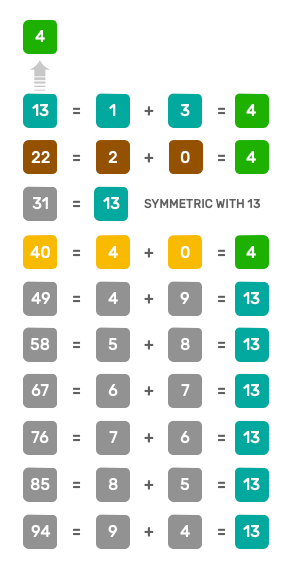Of the ten decompositions identified above, only one concerns the number 22 as well as for number 40 and seven concern the number 13, i.e. 70% of the cases.
In other words number 4 is not always a square

#### Master number 22

The number 4 can only be obtained in three ways:

• 1 + 3 = 4
• 2 + 2 = 4
• 4 + 0 = 4

Decomposition 2 + 2 is as rare as decomposition 4 + 0.

Finally the number 13 is the most commune symbol for number 4.

In Geonumerology, we attach importance to the decomposition of all the numbers preceding the 9 reduced numbers. There are only 29 in all. These are the "Source-Numbers" of Geonumerology.

## Numerology Defects

A monolithic and above-ground linear vision

The main difference between numerology and geo-numerology, in addition to its graphical approach, is to adopt a systemic approach where the relationships between numbers are more important than an isolated interpretation.

Geonumerology links psychology to spirituality without falling into the traps of mysticism. Its method is rational and logical because it is based on the rules of arithmetic and sacred geometry, a true criterium of truth.

A notable difference between the two disciplines lies in the fact that numerology interpretation is most often isolated: one number, then another number, whereas the systemic approach used in GeoNumerology is more close to the holistic vision of Pythagoras than to a Cartesian analytical vision.

As its name suggests, it is a question of studying a system. A system is composed of a certain number of elements (here the 9 numbers), arranged within a structure (here a matrix of 9 boxes) and establishing relationships between them (which we will update).

The main tools of systems thinking such as analogical reasoning, isomorphism, graphic language or modeling are strictly the same as those used in GeoNumerology.

The interpretation of most numerologists comes from what I would call "above ground reasoning, without the slightest reference to the structure used to accommodate the first 9 numbers, nor to the links between these 9 numbers.

Most of the available numerical interpretations work like this:

• if your expression number is x, you're like this, call me;
• if your soul urge number is y, you are like this, call me.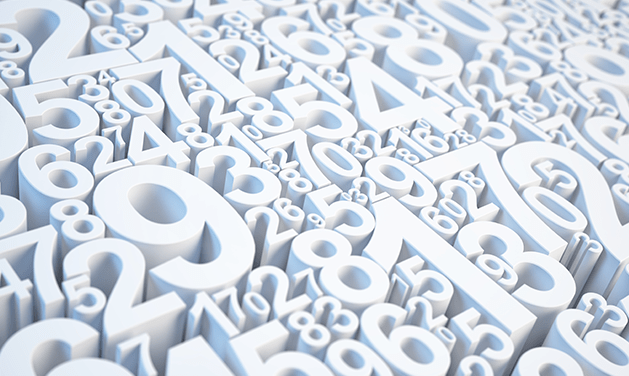We have just seen that the number 4 had three different representations One cannot reasonably interpret in the same way a 4 resulting from 1 + 3, 2 + 2 ou 4 + 0, knowing that the first represents a tetrahedron, the second a cross and the third a square.

The 29 decompositions that precede the 9 reduced numbers are the arithmetic keys that will allow us to do two things :

• Visualize a number in geometric space by adding up one by one the units that comprise it;
• Locate a number and its components in the numeric matrix of the Three Worlds, revealing its role in relation to the whole.

## Reduction & decomposition

The 29 unique ways to get the nine reduced numbers

#### Reduction to 9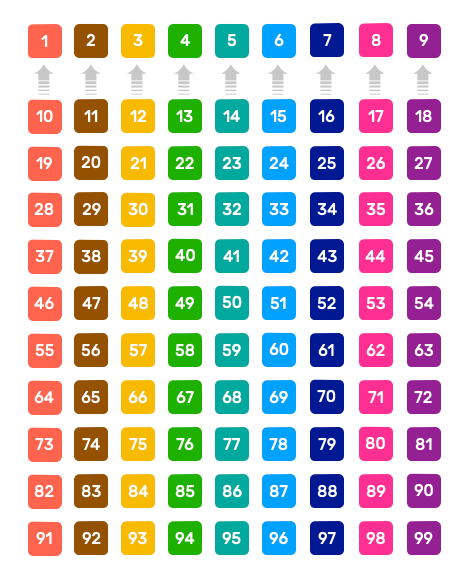All the numbers in a column have the same reduction (indicated in the first row with the first 9 one-digit numbers).
Ex : number 58. Its reduction is 5 + 8  = 13 and 13 = 1 + 3 = 4.

#### Decomposition to 29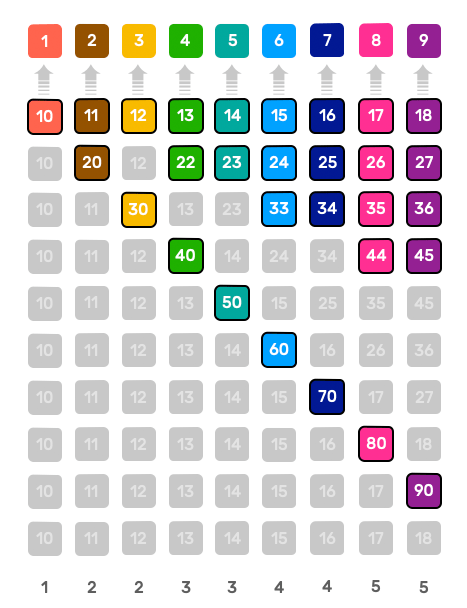Numbers in gray represent duplicates.
We see that apart from the number one all other numbers can be obtained in different ways. The reduced number 4 has three source numbers : 13, 22 & 40.

By studying how all numbers are reduced to the first nine, geonumerology identifies 29 unique possibilities. While all the numbers in a column have the same reduction-number (first row of the table), not all do so in the same way.

We can see that the reduction of a number can be obtained in several ways (except for 1).

If we take the example of the number 7 in the table on the left, we can see that there are four unique possibilities (without the duplicates: grayed cells) to obtain the number 7:

• 1 + 6 (or symmetrical sound 61)
• 2 + 5 (or symmetrical sound 52)
• 3 + 4 (or symmetrical sound 43)
• 7 + 0

## The 29 Source-Numbers

The 29 personality archetypes.

The 29 Source Numbers numbers of geonumerology each correspond to an equation, a formula, which gives us the keys to its geometrical construction : crosses, polygons, solids, figurate numbers, etc.

There are three major types of source numbers:

• Solitary numbers of type X = XO (examples; 1=10, 2=20, 3=30, ...). These numbers correspond to the polygons of the circle;
• Double numbers of type X = YY (examples; 2=11, 4=22, 6=33 and 8=44). These numbers correspond to the double numbers;
• Compound numbers of type X = YZ (examples; 3=12, 4=13, 5=14, etc). Concerns the 15 other numbers named composed numbers.

To know a number, it is first of all to link it to other previous numbers from which it necessarily proceeds, to the conditions which determine its formation beforehand.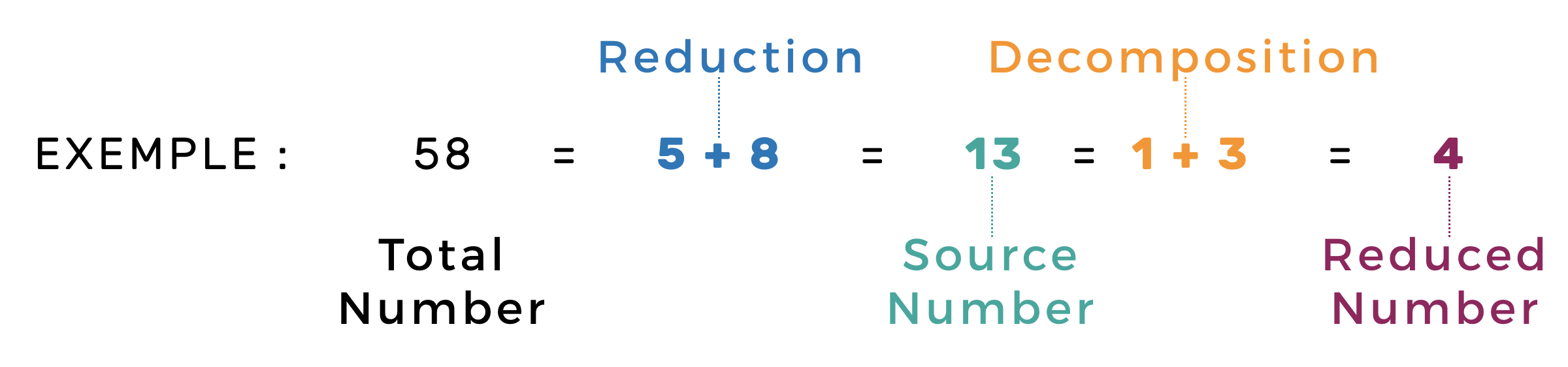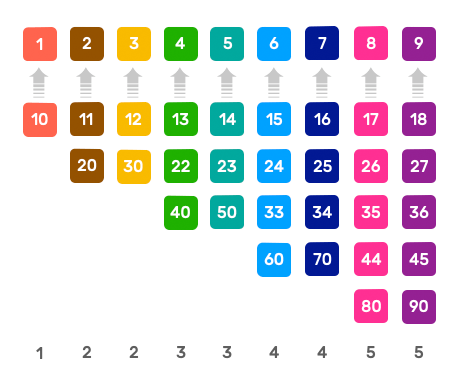In the example on the left, the Number 4 obtained from the total number 58 derives from the source number 13 whose decomposition is 1 + 3.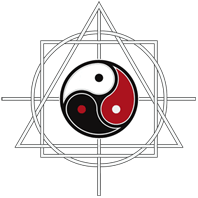THE SACRED GEOMETRY OF YOUR NAME